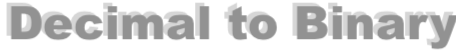How to Convert 5 to BinaryHere we will show you step-by-step how to convert the decimal number 5 to binary.

First, note that decimal numbers use 10 digits (0, 1, 2, 3, 4, 5, 6, 7, 8, and 9) and binary numbers use only 2 digits (0 and 1).

As we explain the steps to converting 5 to binary, it is important to know the name of the parts of a division problem. In a problem like A divided by B equals C, A is the Dividend, B is the Divisor and C is the Quotient.

The Quotient has two parts. The Whole part and the Fractional part. The Fractional part is also known as the Remainder.

Step 1) Divide 5 by 2 to get the Quotient. Keep the Whole part for the next step and set the Remainder aside.

Step 2) Divide the Whole part of the Quotient from Step 1 by 2. Again, keep the Whole part and set the Remainder aside.

Step 3) Repeat Step 2 above until the Whole part is 0.

Step 4) Write down the Remainders in reverse order to get the answer to 5 as a binary.

Here we will show our work so you can follow along:

5 / 2 = 2 with 1 remainder
2 / 2 = 1 with 0 remainder
1 / 2 = 0 with 1 remainder

Then, when we put the remainders together in reverse order, we get the answer. The decimal number 5 converted to binary is therefore:

101

So what we did on the page was to Convert A10 to B2, where A is the decimal number 5 and B is the binary number 101. Which means that you can display decimal number 5 to binary in mathematical terms as follows:

510 = 1012

Decimal to Binary Converter
Need another decimal number as a binary number? How to convert 5 to binary is not all we know. Convert another decimal to binary below:

How to Convert 6 to Binary
Here is our next decimal to binary tutorial on our list.